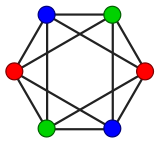# Java Program to Use Color Interchange Method to Perform Vertex Coloring of Graph

• Last Updated : 06 Apr, 2022

Graph Coloring is the method to assign colors to certain elements of a graph. The most common method for this is the Vertex Coloring Method. We will be given m colors, we will apply each color to the vertex. We use here the “Backtracking” Algorithm.Illustration:

Input: V = 4, C = 2

`Here V denotes colour and C denotes color`

Graph:

```{
{ 0, 1, 0, 0, 0, 1, 0, 0, 0, 0 },
{ 1, 0, 1, 0, 0, 0, 1, 0, 0, 0 },
{ 0, 1, 0, 1, 0, 0, 0, 1, 0, 0 },
{ 0, 0, 1, 0, 1, 0, 0, 0, 1, 0 }
};               ```

Output: Solution exists

Colors: 1 2 1 2

Approach:

1. isPossible() method checks if we can keep the color at that particular vertex or not, by checking the colors of the adjacent vertices to ‘v’ have the color ‘c’ that we want to assign to the vertex.
2. Graphcoloring() method is where we try different colors for vertex v, by taking the help of the isPossible() function.
3. The main() function then is just initializing the colors[] array, which has the same usage as with the greedy algorithm, and then calling the graphcoloring() function for vertex 0.
4. When the algorithm is successful it prints the solution, else no solution exists.

Implementation:

Example

siolve

## Java

 `// Java Program to Use Color Interchange Method to` `// Perform Vertex Coloring of Graph`   `// Importing input output classes` `import` `java.io.*;` `// Importing Scanner class to take input from the user` `import` `java.util.Scanner;`   `// Main class` `// GraphColouring` `public` `class` `GFG {`   `    ``// Declaring variables for` `    ``// vertices, Number of colours,` `    ``// colour array, graph array` `    ``private` `int` `vert, numCol;` `    ``private` `int``[] colour;` `    ``private` `int``[][] graph;`   `    ``// Method 1` `    ``// To colour the graph by` `    ``// Taking vertices to be size of graph array` `    ``public` `void` `graphcolour(``int``[][] g, ``int` `c)` `    ``{` `        ``vert = g.length;`   `        ``// Taking the number of colour` `        ``numCol = c;`   `        ``// Taking colour array with size vertices` `        ``colour = ``new` `int``[vert];`   `        ``// Here graph is g` `        ``graph = g;`   `        ``// Now let us implement the try catch exception` `        ``// If the graph is empty it shows no solution`   `        ``// Try block to check for exception` `        ``try` `{`   `            ``solve(``0``);` `            ``System.out.println(``"No solution"``);` `        ``}`   `        ``// Catch block to handle the exception` `        ``catch` `(Exception e) {`   `            ``// If the graph has something` `            ``// It shows solution exists` `            ``System.out.println(``"\nSolution exists "``);`   `            ``// It displays the output` `            ``display();` `        ``}` `    ``}`   `    ``// Method 2` `    ``// To siolve the solve function` `    ``public` `void` `solve(``int` `ve) ``throws` `Exception` `    ``{`   `        ``// If vertices on both the cases are equal` `        ``// Then we print the solution` `        ``if` `(ve == vert)` `            ``throw` `new` `Exception(``"Solution found"``);`   `        ``// Now when we iterate the loop from i till colours.` `        ``for` `(``int` `i = ``1``; i <= numCol; i++) {`   `            ``// Now to check the is Possible function` `            ``// we check if there is any such possible` `            ``// solution if we find one then..` `            ``if` `(isPossible(ve, i)) {`   `                ``// The index of the colour array is set to` `                ``// the index of i and sent for recursion` `                ``// The function again takes solve function` `                ``// and solves until we find all the suitable` `                ``// outcomes Then we set the colour of that` `                ``// index to 0` `                ``colour[ve] = i;` `                ``solve(ve + ``1``);` `                ``colour[ve] = ``0``;` `            ``}` `        ``}` `    ``}`   `    ``// Method 3` `    ``// is Possible function` `    ``public` `boolean` `isPossible(``int` `ve, ``int` `c)` `    ``{`   `        ``// Now it iterates from i till vert` `        ``for` `(``int` `i = ``0``; i < vert; i++)`   `            ``// Now if graph value at any matrix index is` `            ``// equal to 1 and so is the colour equal to the` `            ``// colour index then We return false else true` `            ``if` `(graph[ve][i] == ``1` `&& c == colour[i])` `                ``return` `false``;`   `        ``return` `true``;` `    ``}`   `    ``// Method 4` `    ``// Display function` `    ``public` `void` `display()` `    ``{`   `        ``// Display message only ` `        ``System.out.println(``"\nColours: "``);`   `        ``// Now iterating the ith function` `        ``for` `(``int` `i = ``0``; i < vert; i++)`   `            ``// The colour will be displayed` `            ``System.out.print(colour[i] + ``" "``);`   `        ``// New line` `        ``System.out.println();` `    ``}`   `    ``// Method 5` `    ``// Main driver method` `    ``public` `static` `void` `main(String[] args)` `    ``{`   `        ``// Display message only` `        ``System.out.println(``"Test"``);`   `        ``// Creating an object of main class` `        ``// in the main() method` `        ``GFG gc = ``new` `GFG();`   `        ``// The vertices of the array are 4` `        ``int` `vert = ``4``;`   `        ``// The graph array is declared below` `        ``int``[][] graph` `            ``= { { ``0``, ``1``, ``0``, ``0``, ``0``, ``1``, ``0``, ``0``, ``0``, ``0` `},` `                ``{ ``1``, ``0``, ``1``, ``0``, ``0``, ``0``, ``1``, ``0``, ``0``, ``0` `},` `                ``{ ``0``, ``1``, ``0``, ``1``, ``0``, ``0``, ``0``, ``1``, ``0``, ``0` `},` `                ``{ ``0``, ``0``, ``1``, ``0``, ``1``, ``0``, ``0``, ``0``, ``1``, ``0` `} };`   `        ``// Number of colours totally are 2` `        ``int` `c = ``2``;`   `        ``// Calling the method 1 to colour` `        ``// graph array declared and initialized above` `        ``gc.graphcolour(graph, c);` `    ``}` `}`

Output

```Test

Solution exists

Colours:
1 2 1 2 ```

My Personal Notes arrow_drop_up
Recommended Articles
Page :